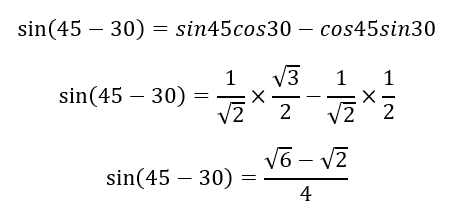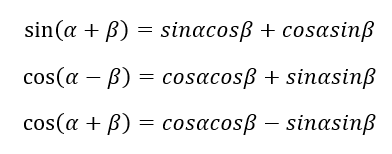# Angle Sums and Differences Worksheets

How to Determine the Sum of Differences with Angles - Finding out the value of the trigonometric identities can be much easier if we use the concept of sum and differences of identities. In other words, it is way tougher to find out the value of sin⁡15, but if we apply a difference identity of the sine function, then it becomes much easier. Like, if we find out the value of sin⁡ (45-30). However, you cannot just write sine 45 and sine 30 separately and subtract them. So, let us discuss the formula in detail. The difference formula for the sine function is sin⁡(α- β) = sinα cosβ - cosα sinβ. In this scenario, α is 45°, while β is 35°. Now, let us solve the problem.Similarly, there are other formulae as well, i.e., sum identity of sine, and both sum and difference identity of cos.• ### Basic Lesson

Guides students solving equations that involve an Angle Sums & Differences Demonstrates answer checking. The positive square root is chosen because Cos 15° lies in Quadrant I. The positive square root is chosen because Cos 5° lies in Quadrant I.

• ### Intermediate Lesson

Demonstrates how to solve more difficult problems.

• ### Independent Practice 1

A really great activity for allowing students to understand the concept of Angle Sums & Differences.

• ### Independent Practice 2

Students find the Angle Sums & Differences in assorted problems. The answers can be found below.

• ### Homework Worksheet

Students are provided with problems to achieve the concepts of Angle Sums & Differences.

• ### Skill Quiz

This tests the students ability to evaluate Angle Sums & Differences.